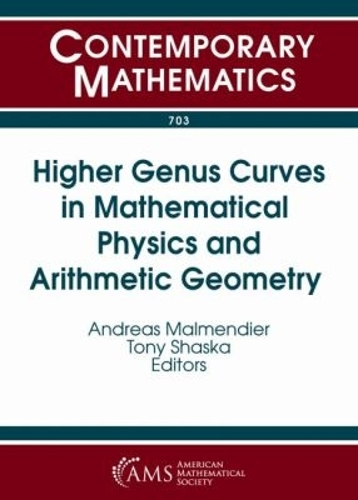•# Higher Genus Curves in Mathematical Physics and Arithmetic Geometry - Contemporary Mathematics (Paperback)

(editor), (editor)
£114.00
Paperback 222 Pages / Published: 30/04/2018
• We can order this

Usually dispatched within 1 week

This volume contains the proceedings of the AMS Special Session on Higher Genus Curves and Fibrations in Mathematical Physics and Arithmetic Geometry, held on January 8, 2016, in Seattle, Washington.

Algebraic curves and their fibrations have played a major role in both mathematical physics and arithmetic geometry. This volume focuses on the role of higher genus curves; in particular, hyperelliptic and superelliptic curves in algebraic geometry and mathematical physics.

The articles in this volume investigate the automorphism groups of curves and superelliptic curves and results regarding integral points on curves and their applications in mirror symmetry. Moreover, geometric subjects are addressed, such as elliptic \$K\$3 surfaces over the rationals, the birational type of Hurwitz spaces, and links between projective geometry and abelian functions.

Publisher: American Mathematical Society
ISBN: 9781470428563
Number of pages: 222
Dimensions: 254 x 178 mm